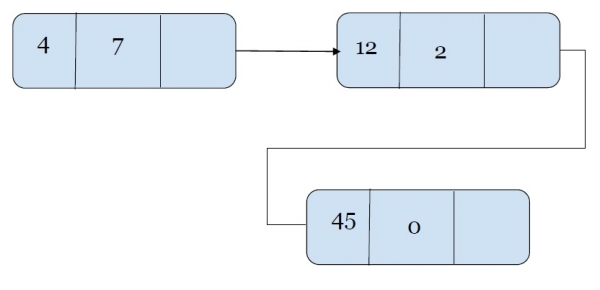To understand this concept better let's first brush up all the basic contents that are required.

linked list is a data structure that stores each element as an object in a node of the list. every note contains two parts data han and links to the next node.

Polynomial is a mathematical expression that consists of variables and coefficients. for example x^2 - 4x + 7

In the Polynomial linked list, the coefficients and exponents of the polynomial are defined as the data node of the list.

For adding two polynomials that are stored as a linked list. We need to add the coefficients of variables with the same power. In a linked list node contains 3 members, coefficient value link to the next node.

a linked list that is used to store Polynomial looks like −

Polynomial : 4x7 + 12x2 + 45This is how a linked list represented polynomial looks like.

Adding two polynomials that are represented by a linked list. We check values at the exponent value of the node. For the same values of exponent, we will add the coefficients.

Example,

Input :
p1= 13x8 + 7x5 + 32x2 + 54
p2= 3x12 + 17x5 + 3x3 + 98

Output : 3x12 + 13x8 + 24x5 + 3x3 + 32x2 + 152

Explanation − For all power, we will check for the coefficients of the exponents that have the same value of exponents and add them. The return the final polynomial.

## Algorithm

Input − polynomial p1 and p2 represented as a linked list.

Step 1: loop around all values of linked list and follow step 2& 3.
Step 2: if the value of a node’s exponent. is greater copy this node to result node and head towards the next node.
Step 3: if the values of both node’s exponent is same add the coefficients and then copy the added value with node to the result.
Step 4: Print the resultant node.

## Example

Live Demo

#include<bits/stdc++.h>
using namespace std;
struct Node{
int coeff;
int pow;
struct Node *next;
};
void create_node(int x, int y, struct Node **temp){
struct Node *r, *z;
z = *temp;
if(z == NULL){
r =(struct Node*)malloc(sizeof(struct Node));
r->coeff = x;
r->pow = y;
*temp = r;
r->next = (struct Node*)malloc(sizeof(struct Node));
r = r->next;
r->next = NULL;
} else {
r->coeff = x;
r->pow = y;
r->next = (struct Node*)malloc(sizeof(struct Node));
r = r->next;
r->next = NULL;
}
}
void polyadd(struct Node *p1, struct Node *p2, struct Node *result){
while(p1->next && p2->next){
if(p1->pow > p2->pow){
result->pow = p1->pow;
result->coeff = p1->coeff;
p1 = p1->next;
}
else if(p1->pow < p2->pow){
result->pow = p2->pow;
result->coeff = p2->coeff;
p2 = p2->next;
} else {
result->pow = p1->pow;
result->coeff = p1->coeff+p2->coeff;
p1 = p1->next;
p2 = p2->next;
}
result->next = (struct Node *)malloc(sizeof(struct Node));
result = result->next;
result->next = NULL;
}
while(p1->next || p2->next){
if(p1->next){
result->pow = p1->pow;
result->coeff = p1->coeff;
p1 = p1->next;
}
if(p2->next){
result->pow = p2->pow;
result->coeff = p2->coeff;
p2 = p2->next;
}
result->next = (struct Node *)malloc(sizeof(struct Node));
result = result->next;
result->next = NULL;
}
}
void printpoly(struct Node *node){
while(node->next != NULL){
printf("%dx^%d", node->coeff, node->pow);
node = node->next;
if(node->next != NULL)
printf(" + ");
}
}
int main(){
struct Node *p1 = NULL, *p2 = NULL, *result = NULL;
create_node(41,7,&p1);
create_node(12,5,&p1);
create_node(65,0,&p1);
create_node(21,5,&p2);
create_node(15,2,&p2);
printf("polynomial 1: ");
printpoly(p1);
printf("\npolynomial 2: ");
printpoly(p2);
result = (struct Node *)malloc(sizeof(struct Node));
}
polynomial 1: 41x^7 + 12x^5 + 65x^0
polynomial after adding p1 and p2 : 41x^7 + 33x^5 + 15x^2 + 65x^0